# Problems for Practice - 4 Notes | Study Algebra for IIT JAM Mathematics - Mathematics

## Mathematics: Problems for Practice - 4 Notes | Study Algebra for IIT JAM Mathematics - Mathematics

The document Problems for Practice - 4 Notes | Study Algebra for IIT JAM Mathematics - Mathematics is a part of the Mathematics Course Algebra for IIT JAM Mathematics.
All you need of Mathematics at this link: Mathematics

For problems 1 & 2 use the Binomial Theorem to expand the given function.

1. Use the Binomial Theorem to expand (4+3x)5.

Solution. Not really a lot to do with this problem.  All we need to do is use the formula from the Binomial Theorem to do the expansion.  Here is that work.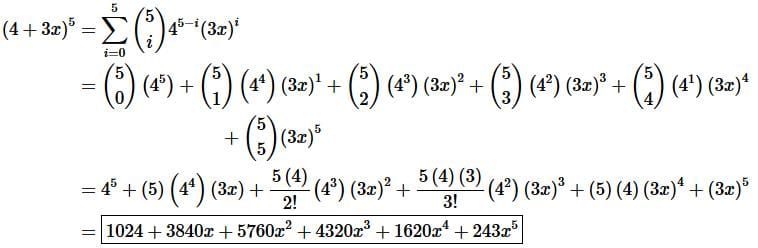2. Use the Binomial Theorem to expand (9−x)4.

Solution. Not really a lot to do with this problem.  All we need to do is use the formula from the Binomial Theorem to do the expansion.  Here is that work.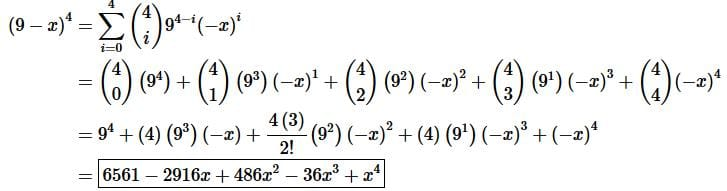3. Write down the first four terms in the binomial series for (1+3x)−6.

Solution. First, we need to make sure it is in the proper form to use the Binomial Series from the notes which in this case we are already in the proper form with k = -6.

Step 2. Now all we need to do is plug into the formula from the notes and write down the first four terms.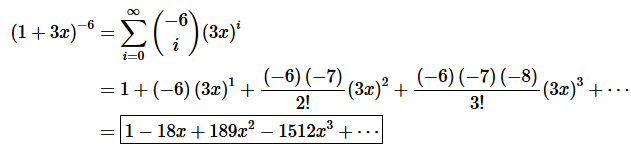4. Write down the first four terms in the binomial series for 3√8−2x.

Solution. First, we need to make sure it is in the proper form to use the Binomial Series.  Here is the proper form for this function,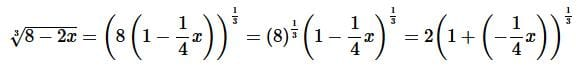Recall that for proper from we need it to be in the form “1+” and so we needed to factor the 8 out of the root and “move” the minus sign into the second term.  Also, as we can see we will have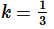Step 2. Now all we need to do is plug into the formula from the notes and write down the first four terms.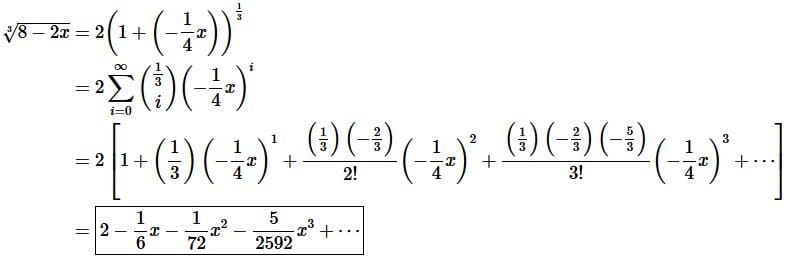The document Problems for Practice - 4 Notes | Study Algebra for IIT JAM Mathematics - Mathematics is a part of the Mathematics Course Algebra for IIT JAM Mathematics.
All you need of Mathematics at this link: MathematicsUse Code STAYHOME200 and get INR 200 additional OFF

## Algebra for IIT JAM Mathematics

182 videos|58 docs

Track your progress, build streaks, highlight & save important lessons and more!

,

,

,

,

,

,

,

,

,

,

,

,

,

,

,

,

,

,

,

,

,

;